# Divide 5

Divide 288 in the following ratio

3 : 4 : 5

Result

a =  72
b =  96
c =  120

#### Solution:Leave us a comment of example and its solution (i.e. if it is still somewhat unclear...):

Showing 0 comments:Be the first to comment!## Next similar examples:

1. 1.5 divided1.5 divided by 1 = w divided by 4
2. Louvers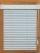The company charges for office equipment louvers total 262 Eur. From the delivery bill is obvious that the louvers are 76 Eur expensive than their installation. What percentage of the total charged is the installation of louvers?
3. Ratio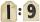Unfold number 1963 in the ratio 9:2:2.
4. Arble bagA marble bag sold by Rachel's Marble Company contains 5 orange marbles for every 6 green marbles. If a bag has 35 orange marbles, how many green marbles does it contain?
5. DonutsFind how many donuts each student will receive if you share 126 donuts in a ratio of 1:5:8
6. Two numbersFind two numbers whose difference and ratio is 2.
7. Percent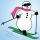From 99 children who participated in the ski course were 23 excellent skiers, 13 good and 20 average and the rest were beginners. Calculate this data in percentages.
8. 100 %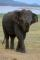How much is 100%, if 17 % is 1169?
9. Shirt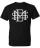Mrs. Vítková bought each of his three children the same shirt paid CZK 1,000. Saleswoman she returned 568,60 CZK. What was the price of one shirt?
10. Bulbs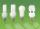In the box are 6 bulbs with power 75 W, 14 bulbs with power 40 W and 15 with 60 W. Calculate probability that a randomly selected bulb is:
11. Percents - easyHow many percent is 432 out of 434?
12. Down syndromeDown syndrome is one of the serious diseases caused by a gene mutation. Down syndrome occurs in approximately every 550-born child. Express the incidence of Down's syndrome in newborns at per mille.
13. Conference148 is the total number of employees. The conference was attended by 22 employees. How much is it in percent?
14. Apples 2James has 13 apples. He has 30 percent more apples than Sam. How many apples has Sam?
15. New refrigeratorNew refrigerator sells for 1024 USD, Monday will be 25% discount. How much USD will save, and what will be the price?
16. Base, percents, valueBase is 344084 which is 100 %. How many percent is 384177?
17. Sales offGoods is worth € 70 and the price of goods fell two weeks in a row by 10%. How many % decreased overall?